﻿ 基于参数估计的海面运动舰船SAR成像方法
«上一篇文章快速检索 高级检索

 雷达学报2016, Vol. 5Issue (3): 326-332  DOI: 10.12000/JR151040

### 引用本文 [复制中英文]

[复制中文]
Yun Yajiao, Qi Xiangyang and Li Ning. Moving ship SAR imaging based on parameter estimation[J]. Journal of Radars, 2016, 5(3): 326-332. DOI: 10.12000/JR15104.
[复制英文]

### 文章历史

(中国科学院电子学研究所 北京 100190)
(中国科学院大学 北京 100039)

Moving Ship SAR Imaging Based on Parameter Estimation
(Institute of Electronics, Chinese Academy of Sciences, Beijing 100190, China)
(University of Chinese Academy of Sciences, Beijing 100039, China)
Foundation Item: The National Ministries Foundation
Abstract: The Doppler parameters of moving targets affect the conventional Synthetic Aperture Radar (SAR) imaging. In this study, the relation between the motion and Doppler parameters is established. With improved popular technology, a set of moving ship SAR imaging processes is proposed to obtain a focused and rightlocated image. Simulations and experimental data are used to verify the method.
Key words: Synthetic Aperture Radar (SAR)     Moving target     Maritime ship imagining
1 引言

2 运动目标信号分析

SAR回波信号经过检波和距离压缩后，可表示为：
 $s(t,\tau ) = \sigma \exp \left( { - {\rm{j2}}\pi {f_{\rm{0}}}\tau } \right)$ (1)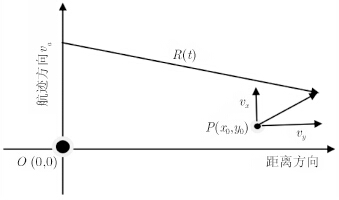图 1 SAR几何模型 Fig. 1 The geometric model of SAR

 $R(t) = \sqrt {{{({y_0} + {v_y}t)}^2} + {{({x_0} + {v_x}t - {v_{\rm{a}}}t)}^2}}$ (2)

 $\begin{array}{*{20}{l}} {R(t - {t_{\rm{c}}}) = {y_{\rm{c}}} + {v_y}(t - {t_{\rm{c}}})}\\ {\;\;\;\;\;\;\;\;\;\;\;\;\;\;\; + \frac{{{{({v_{\rm{a}}} - {v_x})}^2}}}{{2{y_{\rm{c}}}}}{{(t - {t_{\rm{c}}})}^2}} \end{array}$ (3)

 $\begin{array}{*{20}{l}} {s\left( {t - {t_{\rm{c}}},\tau } \right) = \sigma \exp \left\{ { - {\rm{j}}\frac{{{\rm{4}}\pi }}{\lambda }\left[{{y_{\rm{c}}} + {v_y}\left( {t - {t_{\rm{c}}}} \right)} \right.} \right.}\\ {\left. {\left. {\;\;\;\;\;\;\;\;\;\;\;\;\;\;\;\;\;\; + \frac{{{{\left( {{v_{\rm{a}}} - {v_x}} \right)}^2}}}{{2{y_{\rm{c}}}}}{{\left( {t - {t_{\rm{c}}}} \right)}^2}} \right]} \right\}} \end{array}$ (4)

 $f = - \frac{{\rm{2}}}{\lambda }\left[{{v_y} + \frac{{{{({v_{\rm{a}}} - {v_x})}^2}}}{{{y_{\rm{c}}}}}(t - {t_{\rm{c}}})} \right]$ (5)

3 多普勒参数与运动目标速度的估计

3.1 多普勒中心频率与径向速度的估计

 $M = {\rm{round}}\left( {\frac{{{f_{\rm{e}}} - {{f'}_{{\rm{tc}}}}}}{{{\rm{PRF}}}}} \right)$ (6)

 ${f_{{\rm{tc}}}} = {f'_{{\rm{tc}}}} + M \times {\rm{PRF}}$ (7)

 $\Delta R(t - {t_{\rm{c}}}) = {v_y}(t - {t_{\rm{c}}}) + \frac{{{{({v_{\rm{a}}} - {v_x})}^2}}}{{2{y_{\rm{c}}}}}{(t - {t_{\rm{c}}})^2}$ (8)

3.2 多普勒调频率与方位向速度的估计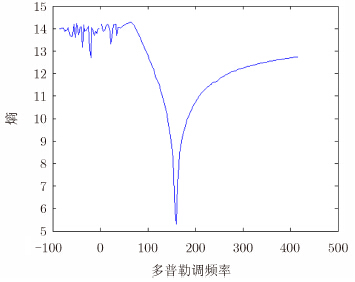图 2 不同调频率对应的熵 Fig. 2 The entropy of different Doppler frequency rate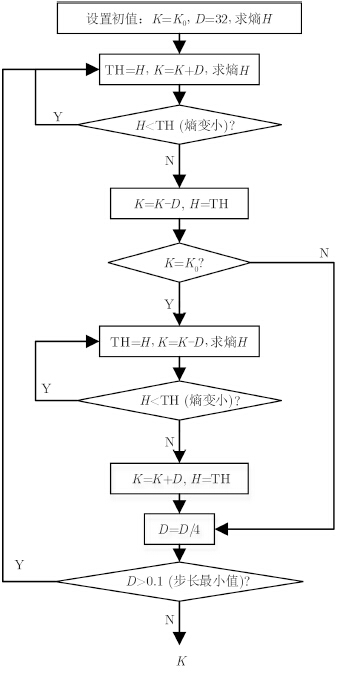图 3 调频率估计流程 Fig. 3 Flow chart of Doppler frequency rate estimation
4 算法流程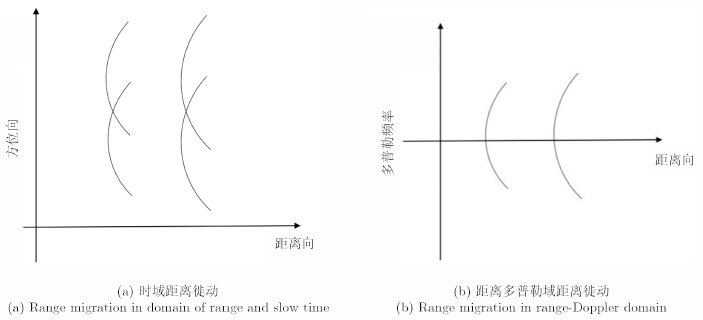图 4 距离徙动 Fig. 4 Range migration

 $\begin{array}{*{20}{l}} {\Delta {R_t}({f_{\rm{a}}}) = \frac{{{\lambda ^2}{y_{\rm{c}}}}}{{{\rm{8}}{{({v_{\rm{a}}} - {v_x})}^2}}}f_{\rm{a}}^2 - \frac{{\lambda {y_{\rm{c}}}{v_y}}}{{{\rm{2}}{{({v_{\rm{a}}} - {v_x})}^2}}}{f_{\rm{a}}}}\\ {\;\;\;\;\;\;\;\;\;\;\;\; = \frac{{{\lambda ^2}{y_{\rm{c}}}}}{{{\rm{8}}{{({v_{\rm{a}}} - {v_x})}^2}}}f_{\rm{a}}^2 - \frac{{\lambda {y_{\rm{c}}}{v_y}}}{{{\rm{2}}{{({v_{\rm{a}}} - {v_x})}^2}}}{f_{\rm{a}}}}\\ {\;\;\;\;\;\;\;\;\;\;\;\;\;\;\; + \frac{{{y_{\rm{c}}}{v_y}^2}}{{2{{({v_{\rm{a}}} - {v_x})}^2}}} - \frac{{{y_{\rm{c}}}{v_y}^2}}{{2{{({v_{\rm{a}}} - {v_x})}^2}}}}\\ {\;\;\;\;\;\;\;\;\;\;\;\; = \frac{{{\lambda ^2}{y_{\rm{c}}}}}{{8{{({v_{\rm{a}}} - {v_x})}^2}}}{{({f_{\rm{a}}} - {f_{{\rm{tc}}}})}^2} - \frac{{{y_{\rm{c}}}v_{\rm{y}}^2}}{{2{{({v_{\rm{a}}} - {v_x})}^2}}}} \end{array}$ (9)

5 仿真与实测数据成像结果及分析表 1 仿真参数 Tab. 1 Simulation parameters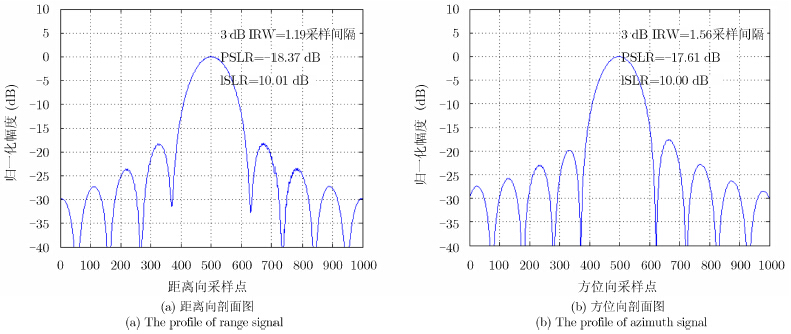图 5 点目标信号质量分析 Fig. 5 Quality analysis of point target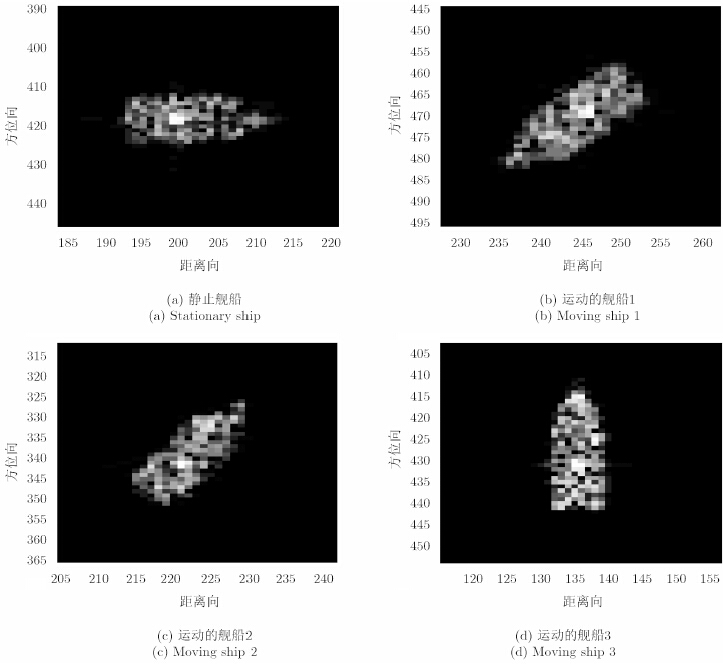图 6 本文算法成像效果 Fig. 6 The results by using this article imaging algorithm表 2 动目标的参数估计结果 Tab. 2 The estimation results of moving target parameters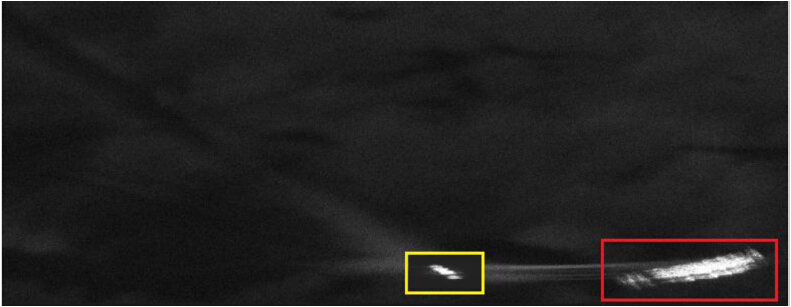图 7 实测数据处理结果 Fig. 7 The imaging of measured data

6 结论

  Ausherman D A, Kozma A, Walker J L, et al. Developments in radar imaging[J]. IEEE Transactions on Aerospace and Electronic Systems, 1984, AES-20(4): 363-400.(1)  张澄波. 综合孔径雷达: 原理、系统分析与应用[M]. 北京: 科学出版社, 1989. Zhang Cheng-bo. Synthetic Aperture Radar: Theory, System Analysis and Application[M]. Beijing: Science Press, 1989.(1)  Chen V C and Baolun L. Hybird SAR/ISAR for distributed ISAR imaging of moving targerts[C]. 2015 IEEE Radar Conference (RadarCon), Arlington, 2015: 0658-0663.(1)  Noviellp C, Fomaro G, and Martorella M. Focused SAR image formation of moving targets based on Doppler parameter estimation[J]. IEEE Transactions on Geoscience and Remote Sensing, 2015, 53(6): 3460-3470.(1)  Freeman A and Currie A. Synthetic Aperture Radar (SAR) images of moving targets[J]. GEC Journal of Research, 1987, 17(3): 629-635.(1)  Raney R K. Synthetic aperture imaging radar and moving targets[J]. IEEE Transactions on Aerospace and Electronic Systems, 1971, AES-7(3): 499-505.(1)  Barbarossa S. Detection and imaging of moving objects with synthetic aperture radar Part 1: Optimal detection and parameter estimation theory[J]. IEE Proceedings F Radar and Signal Processing, 1992, 139(1): 79-88.(1)  Klemm R. Application of Space-time Adaptive Processing[M]. The Institution of Electrical Engineers, 2004.(1)  Perry R P, DiPietro R C, and Fante R. SAR imaging of moving targets[J]. IEEE Transactions on Aerospace and Electronic Systems, 1999, 35(1): 188-200.(1)  师君, 马龙, 韦顺军, 等. 基于导航数据的Ka波段InSAR成像处理与分析[J]. 雷达学报, 2014, 3(1): 19-27. Shi Jun, Ma Long, Wei Shun-jun, et al. Ka-band InSAR imaging and analysis based on IMU data[J]. Journal of Radars, 2014, 3(1): 19-27.(1)  Martorella M, Pastina D, Berizzi F, et al. Spaceborne radar imaging of maritime moving targets with the Cosmo-SkyMed SAR system[J]. IEEE Journal of Selected Topics in Applied Earth Observations and Remote Sensing, 2014, 7(7): 2797-2810.(1)  汪玲, 朱岱寅, 朱兆达. 基于SAR实测数据的舰船成像研究[J]. 电子与信息学报, 2007, 29(2): 401-404. Wang Ling, Zhu Dai-yin, and Zhu Zhao-da. Research on ship imaging based on the real data of SAR[J]. Journal of Electronics & Information Technology, 2007, 29(2): 401-404.(1)  邢孟道, 保铮. 外场实测数据的舰船目标ISAR成像[J]. 电子与信息学报, 2001, 23(12): 1271-1277. Xing Meng-dao and Bao Zheng. The measured data of ship ISAR imaging[J]. Journal of Electronics & Information Technology, 2001, 23(12): 1271-1277.(1)  Musman S, Kerr D, and Bachmann C. Automatic recognition of ISAR ship images[J]. IEEE Transactions on Aerospace & Electronic Systems, 1996, 32(4): 1392-1404.(1)  Bamler R. Doppler frequency estimation and the Cramer-Rao bound[J]. IEEE Transactions on Geoscience and Remote Sensing, 1991, 29(3): 385-390.(1)  Xue F, Chen Q, and Zhang Y. A Doppler frequency estimation algorithm based on band mismatch[C]. 2014 IEEE International Conference on Orange Technologies (ICOT), Xi’an, 2014: 77-80.(1)  邢孟道, 保铮. 基于运动参数估计的SAR成像[J]. 电子学报, 2001, 29(12A): 1824-1828. Xing Meng-dao and Bao Zheng. SAR imaging motion based on parameter estimation[J]. Acta Electronica Sinica, 2001, 29(12A): 1824-1828.(1)  Chen C C and Candrews H. Target-Motion-Induced radar imaging[J]. IEEE Transactions on Aerospace and Electronic Systems, 1980, AES-16(1): 2-14.(1)  Li X, Liu G, and Ni J. Autofocusing of ISAR images based on entropy minimization[J]. IEEE Transactions on Aerospace and Electronic Systems, 1999, 35(4): 1240-1252.(1)  Wang J and Liu X. Automatic correction of range migration in SAR imaging[J]. IEEE Geoscience and Remote Sensing Letters, 2010, 7(2): 256-260.(1)  Li F K, Held D N, Curlander J C, et al. Doppler parameter estimation for spaceborne Synthetic-Aperture radars[J]. IEEE Transactions on Geoscience and Remote Sensing, 1985, 23(1): 47-56.(1)  Moreira J R. A new method of aircraft motion error extraction from radar raw data for real time motion compensation[J]. IEEE Transactions on Geoscience and Remote Sensing, 1990, 28(4): 620-626.(1)  Wang J and Liu X. SAR Minimum-Entropy autofocus using an Adaptive-Order polynomial model[J]. IEEE Geosciences and Remote Sensing Letters, 2006, 3(4): 512-516.(1)